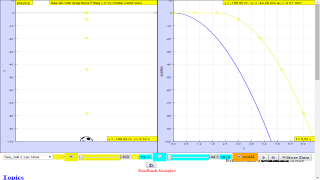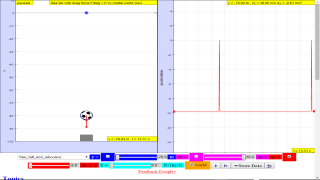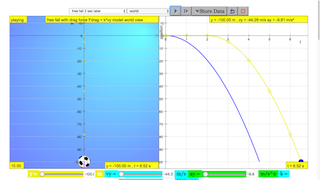## Topics

Kinematics
Speed, velocity and acceleration
Graphical analysis of motion
Free-fall
Effect of air resistance

## Description

This simulation has a drop-down menu for exploration of
(i) at rest  use of progressive mathematical model is encouraged Y = 0 for example
(ii) moving with uniform velocity, use of progressive mathematical model is encouraged
(iii) moving with non-uniform velocity (eg, constant acceleration) use of progressive mathematical model is encouraged
When only the  velocity-time graph check-box is selected, it can be explored for the following cases too.
(i) at rest
(ii) moving with uniform velocity (eg, no acceleration) model of the form Y = Y0+u*t
(iii) moving with uniform acceleration (eg, constant acceleration = 9.81 m/s^2) model of the form Y = Y0+u*t+0.5*g*t
(iv) moving with non-uniform acceleration (eg, with small ot large drag force acting thus acceleration changes).
The default acceleration is set at-9.81 m/s^2 which is near to the Earth is constant and is approximately 10 m/s 2.
Lastly, by  selecting the 3 options of
"free fall"
"free_fall_with_small_air_resistance"
"free_fall_with_large_air_resistance"
It can provide the experience and evidences for describing the motion of bodies with constant weight falling with (large and small) or without air resistance, including reference to terminal velocity, a constant velocity as a result of balanced forces of weight of mass and the drag force giving rise to zero acceleration.

## Sample Learning Goals

(e) plot and interpret a displacement-time graph and a velocity-time graph
(f) deduce from the shape of a displacement-time graph when a body is:
(i) at rest
(ii) moving with uniform velocity
(iii) moving with non-uniform velocity
(g) deduce from the shape of a velocity-time graph when a body is:
(i) at rest
(ii) moving with uniform velocity
(iii) moving with uniform acceleration
(iv) moving with non-uniform acceleration
(i) state that the acceleration of free fall for a body near to the Earth is constant and is approximately 10 m/s 2
(j) describe the motion of bodies with constant weight falling with or without air resistance, including reference to terminal velocity

## Version:

Intro Page

ball taken from: http://psychology.wikia.com/wiki/File:Soccer_ball.svg

http://weelookang.blogspot.sg/2013/12/ejss-free-fall-model.html

### For Teachers

ForTeacher

Teacher-Submitted Activities

 Title Authors Level Type Updated

Share an activity! email

### Translations

Code Language Translator Run### Software Requirements

SoftwareRequirements

 Android iOS Windows MacOS with best with Chrome Chrome Chrome Chrome support full-screen? Yes. Chrome/Opera No. Firefox/ Samsung Internet Not yet Yes Yes cannot work on some mobile browser that don't understand JavaScript such as..... cannot work on Internet Explorer 9 and below

### CreditsThis email address is being protected from spambots. You need JavaScript enabled to view it.

### end faq

http://iwant2study.org/ospsg/index.php/interactive-resources/physics/02-newtonian-mechanics/01-kinematics/346-freefall01pri

### Worksheet

1. Motion in One Dimension student worksheet (dl-gwf) Link1Link2  by Lyna, Gavin, Dave and lookang

### Examples

From Dave and Gavin,

1.A sky diver jumps out of a hovering helicopter. A 2 seconds afterwards another sky diver jumps out and they both fall along the same vertical line. Ignore air resistance so that both sky divers fall with the same acceleration.
a.Does the difference in their speeds stay the same throughout the fall?

suggested answer: after t =2 s, the difference is always the same difference as shown by the constant difference.

b.Does the vertical distance between them stay the same throughout the fall?

suggested answer: after t =2 s, the difference in position increases as shown by the increasing difference of YELLOW minus BLUE line.

Question 2

2.A tennis ball thrown from the top of a building is given an initial velocity of 20 m/s straight upward. The building is 50 m high, and the ball just misses the edge of the roof on its way down. Using t = 0 as the time the ball leaves the thrower’s hand, determine
a.the time at which the ball reaches its maximum height

suggested answer: t = 2.0 s

b.the maximum height

suggested answer: H maximum = 70.4 m

c.the time at which the ball returns to the height from which it was thrown

suggested answer: t = 4.0 or 4.1 s

d.the velocity of the ball at this instant

suggested answer: v = -20.2 m/s

e.the velocity and position of the ball at t = 5 s

suggested answer: y = 27.4 m and v = -29.1 m/s

### Versions

2. http://weelookang.blogspot.sg/2014/03/ejss-kinematics-in-y-model.html Blogpost on JavaScript version by Loo Kang Wee
3. Blogpost on JavaScript version by Loo Kang Wee
4. http://weelookang.blogspot.sg/2013/12/ejss-kinematics-model.html template objects from lookang
5. http://weelookang.blogspot.sg/2010/09/ejs-open-source-bouncing-ball-with-drag.html Blogpost on Java vesion by Loo Kang Wee and Fu-Kwun Hwang
6. http://weelookang.blogspot.sg/2010/06/ejs-open-source-kinematics-java-applet.html
7. http://www.compadre.org/osp/items/detail.cfm?ID=13050 Physlet One-Dimensional Kinematics Illustrations Package by Andreu Glasmann, Wolfgang Christian, and Mario Belloni

### Other Resources

1. http://www.phy.ntnu.edu.tw/ntnujava/index.php?topic=421.0 Elastic bouncing ball Java Version by Fu-Kwun Hwang
2. http://butikov.faculty.ifmo.ru/Projects/Falling_bodies.html#_applet Falling Object Java 3D version by Eugene Butikov

### Other Hands-on kit

1. https://sites.google.com/site/iprofmeca/session-1-free-fall by This email address is being protected from spambots. You need JavaScript enabled to view it.
2. http://www.addest.com/products/category/Science_Kits by Addest TechnovationKinematics Kit
3. http://www.addest.com/products/category/Science_Kits by Addest TechnovationFree Fall Kit

### Project related:

Understanding Teacher Learning Community as Support for Implementation of Open Source Physics for Conceptual Instruction
Project Number: OER 10/15 GWF
Project Duration: 01 July 2015 - 30 April 2017

### Family of Resources

Secondary  Junior College## Topics

Kinematics
Speed, velocity and acceleration
Graphical analysis of motion
Free-fall
Effect of air resistance

## Description

This simulation has a drop-down menu for exploration of
(i) at rest  use of progressive mathematical model is encouraged Y = 0 for example
(ii) moving with uniform velocity, use of progressive mathematical model is encouraged
(iii) moving with non-uniform velocity (eg, constant acceleration) use of progressive mathematical model is encouraged
When only the  velocity-time graph check-box is selected, it can be explored for the following cases too.
(i) at rest
(ii) moving with uniform velocity (eg, no acceleration) model of the form Y = Y0+u*t
(iii) moving with uniform acceleration (eg, constant acceleration = 9.81 m/s^2) model of the form Y = Y0+u*t+0.5*g*t
(iv) moving with non-uniform acceleration (eg, with small ot large drag force acting thus acceleration changes).
The default acceleration is set at-9.81 m/s^2 which is near to the Earth is constant and is approximately 10 m/s 2.
Lastly, by  selecting the 3 options of
"free fall"
"free_fall_with_small_air_resistance"
"free_fall_with_large_air_resistance"
It can provide the experience and evidences for describing the motion of bodies with constant weight falling with (large and small) or without air resistance, including reference to terminal velocity, a constant velocity as a result of balanced forces of weight of mass and the drag force giving rise to zero acceleration.

## Sample Learning Goals

(e) plot and interpret a displacement-time graph and a velocity-time graph
(f) deduce from the shape of a displacement-time graph when a body is:
(i) at rest
(ii) moving with uniform velocity
(iii) moving with non-uniform velocity
(g) deduce from the shape of a velocity-time graph when a body is:
(i) at rest
(ii) moving with uniform velocity
(iii) moving with uniform acceleration
(iv) moving with non-uniform acceleration
(i) state that the acceleration of free fall for a body near to the Earth is constant and is approximately 10 m/s 2
(j) describe the motion of bodies with constant weight falling with or without air resistance, including reference to terminal velocity

## Version:

Intro Page

ball taken from: http://psychology.wikia.com/wiki/File:Soccer_ball.svg

http://weelookang.blogspot.sg/2013/12/ejss-free-fall-model.html

### For Teachers

ForTeacher

Teacher-Submitted Activities

 Title Authors Level Type Updated

Share an activity! email

### Translations

Code Language Translator Run### Software Requirements

SoftwareRequirements

 Android iOS Windows MacOS with best with Chrome Chrome Chrome Chrome support full-screen? Yes. Chrome/Opera No. Firefox/ Samsung Internet Not yet Yes Yes cannot work on some mobile browser that don't understand JavaScript such as..... cannot work on Internet Explorer 9 and below

### CreditsThis email address is being protected from spambots. You need JavaScript enabled to view it.

### end faq## Topics

Kinematics
Speed, velocity and acceleration
Graphical analysis of motion
Free-fall
Effect of air resistance

## Description

This simulation has a drop-down menu for exploration of
(i) at rest  use of progressive mathematical model is encouraged Y = 0 for example
(ii) moving with uniform velocity, use of progressive mathematical model is encouraged
(iii) moving with non-uniform velocity (eg, constant acceleration) use of progressive mathematical model is encouraged
When only the  velocity-time graph check-box is selected, it can be explored for the following cases too.
(i) at rest
(ii) moving with uniform velocity (eg, no acceleration) model of the form Y = Y0+u*t
(iii) moving with uniform acceleration (eg, constant acceleration = 9.81 m/s^2) model of the form Y = Y0+u*t+0.5*g*t
(iv) moving with non-uniform acceleration (eg, with small ot large drag force acting thus acceleration changes).
The default acceleration is set at-9.81 m/s^2 which is near to the Earth is constant and is approximately 10 m/s 2.
Lastly, by  selecting the 3 options of
"free fall"
"free_fall_with_small_air_resistance"
"free_fall_with_large_air_resistance"
It can provide the experience and evidences for describing the motion of bodies with constant weight falling with (large and small) or without air resistance, including reference to terminal velocity, a constant velocity as a result of balanced forces of weight of mass and the drag force giving rise to zero acceleration.

## Sample Learning Goals

(e) plot and interpret a displacement-time graph and a velocity-time graph
(f) deduce from the shape of a displacement-time graph when a body is:
(i) at rest
(ii) moving with uniform velocity
(iii) moving with non-uniform velocity
(g) deduce from the shape of a velocity-time graph when a body is:
(i) at rest
(ii) moving with uniform velocity
(iii) moving with uniform acceleration
(iv) moving with non-uniform acceleration
(i) state that the acceleration of free fall for a body near to the Earth is constant and is approximately 10 m/s 2
(j) describe the motion of bodies with constant weight falling with or without air resistance, including reference to terminal velocity

## Version:

### Translations

Code Language Translator Run### Software Requirements

SoftwareRequirements

 Android iOS Windows MacOS with best with Chrome Chrome Chrome Chrome support full-screen? Yes. Chrome/Opera No. Firefox/ Samsung Internet Not yet Yes Yes cannot work on some mobile browser that don't understand JavaScript such as..... cannot work on Internet Explorer 9 and below

### CreditsThis email address is being protected from spambots. You need JavaScript enabled to view it.; Francisco Esquembre; Félix J. García

### end faq